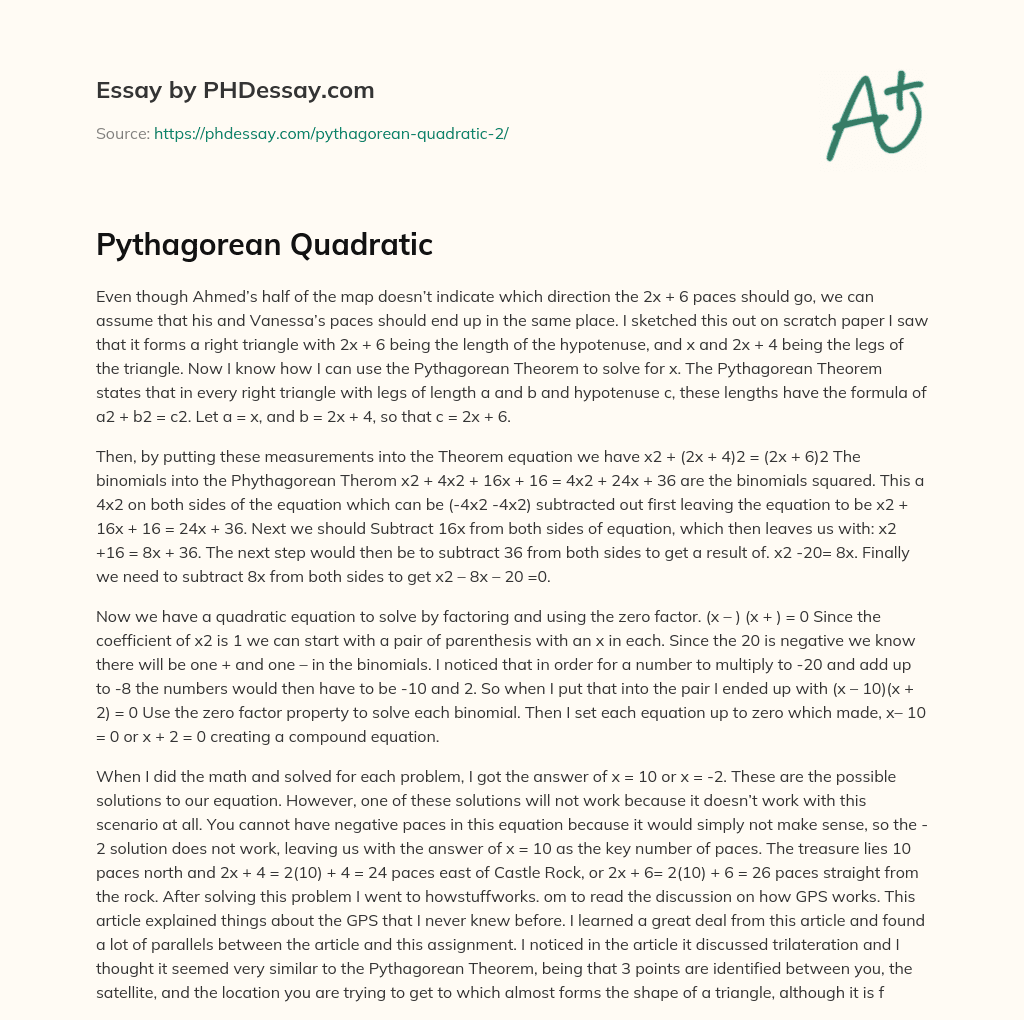Last Updated 28 Jan 2021

Words 790 (3 pages)
7 views

Even though Ahmed’s half of the map doesn’t indicate which direction the 2x + 6 paces should go, we can assume that his and Vanessa’s paces should end up in the same place. I sketched this out on scratch paper I saw that it forms a right triangle with 2x + 6 being the length of the hypotenuse, and x and 2x + 4 being the legs of the triangle. Now I know how I can use the Pythagorean Theorem to solve for x. The Pythagorean Theorem states that in every right triangle with legs of length a and b and hypotenuse c, these lengths have the formula of a2 + b2 = c2. Let a = x, and b = 2x + 4, so that c = 2x + 6.

Then, by putting these measurements into the Theorem equation we have x2 + (2x + 4)2 = (2x + 6)2 The binomials into the Phythagorean Therom x2 + 4x2 + 16x + 16 = 4x2 + 24x + 36 are the binomials squared. This a 4x2 on both sides of the equation which can be (-4x2 -4x2) subtracted out first leaving the equation to be x2 + 16x + 16 = 24x + 36. Next we should Subtract 16x from both sides of equation, which then leaves us with: x2 +16 = 8x + 36. The next step would then be to subtract 36 from both sides to get a result of. x2 -20= 8x. Finally we need to subtract 8x from both sides to get x2 – 8x – 20 =0.

Now we have a quadratic equation to solve by factoring and using the zero factor. (x – ) (x + ) = 0 Since the coefficient of x2 is 1 we can start with a pair of parenthesis with an x in each. Since the 20 is negative we know there will be one + and one – in the binomials. I noticed that in order for a number to multiply to -20 and add up to -8 the numbers would then have to be -10 and 2. So when I put that into the pair I ended up with (x – 10)(x + 2) = 0 Use the zero factor property to solve each binomial. Then I set each equation up to zero which made, x– 10 = 0 or x + 2 = 0 creating a compound equation.

Order custom essay Pythagorean Quadratic with free plagiarism report

GET ORIGINAL PAPER

When I did the math and solved for each problem, I got the answer of x = 10 or x = -2. These are the possible solutions to our equation. However, one of these solutions will not work because it doesn’t work with this scenario at all. You cannot have negative paces in this equation because it would simply not make sense, so the -2 solution does not work, leaving us with the answer of x = 10 as the key number of paces. The treasure lies 10 paces north and 2x + 4 = 2(10) + 4 = 24 paces east of Castle Rock, or 2x + 6= 2(10) + 6 = 26 paces straight from the rock. After solving this problem I went to howstuffworks. om to read the discussion on how GPS works. This article explained things about the GPS that I never knew before. I learned a great deal from this article and found a lot of parallels between the article and this assignment. I noticed in the article it discussed trilateration and I thought it seemed very similar to the Pythagorean Theorem, being that 3 points are identified between you, the satellite, and the location you are trying to get to which almost forms the shape of a triangle, although it is far more complex than that and is using circles instead of triangles.

However the way I saw it is that my position and where I am trying to go would be the legs and the satellite that is directing me to my location from my current position, would have to be a 450 angle, making it the hypotenuse. If one was able to put that into numbers or do some calculate the distance of the satellite, themselves, one would easily be able to calculate your distance using the Pythagorean Theorem.

Using this information a GPS is able to detect where you are, how far you are away from your location and the time it will take to get there, which can be incredibly handy, especially for those who do not have the greatest since of direction. After reading this article it should be that the Pythagorean Theorem is much more useful than I thought. It really simplifies the use of maps and a GPS system. It can be incredibly helpful in many aspects of everyday life.This essay was written by a fellow student. You can use it as an example when writing your own essay or use it as a source, but you need cite it.

## Get professional help and free up your time for more important courses

Starting from 3 hours delivery 450+ experts on 30 subjects
get essay help 124  experts online

Did you know that we have over 70,000 essays on 3,000 topics in our database?

Explore how the human body functions as one unit in harmony in order to life